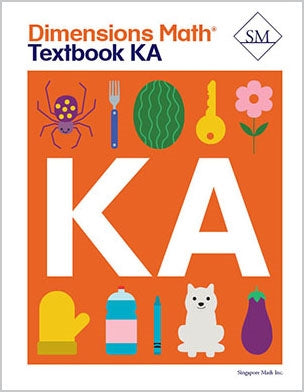# Dimensions Math Textbook KA

\$ 12.00

Dimensions Math® Textbook KA

Chapter 1: Match, Sort, and Classify

Lesson 1: Left and Right
Lesson 2: Same and Similar
Lesson 3: Look for One That is Different
Lesson 4: How Does it Feel?
Lesson 5: Match the Things That Go Together
Lesson 6: Sort
Lesson 7: Practice

Chapter 2: Numbers to 5

Lesson 1: Count to 5
Lesson 2: Count Things up to 5
Lesson 3: Recognize the Numbers 1 to 3
Lesson 4: Recognize the Numbers 4 and 5
Lesson 5: Count and Match
Lesson 6: Write the Numbers 1 and 2
Lesson 7: Write the Number 3
Lesson 8: Write the Number 4
Lesson 9: Trace and Write 1 to 5
Lesson 10: Zero
Lesson 11: Picture Graphs
Lesson 12: Practice

Chapter 3: Numbers to 10

Lesson 1: Count 1 to 10
Lesson 2: Count Up to 7 Things
Lesson 3: Count Up to 9 Things
Lesson 4: Count Up to 10 Things — Part 1
Lesson 5: Count Up to 10 Things — Part 2
Lesson 6: Recognize the Numbers 6 to 10
Lesson 7: Write the Numbers 6 and 7
Lesson 8: Write the Numbers 8, 9, and 10
Lesson 9: Write the Numbers 6 to 10
Lesson 10: Count and Write the Numbers 1 to 10
Lesson 11: Ordinal Positions
Lesson 12: One More Than
Lesson 13: Practice

Chapter 4: Shapes and Solids

Lesson 1: Curved or Flat
Lesson 2: Solid Shapes
Lesson 3: Closed Shapes
Lesson 4: Rectangles
Lesson 5: Squares
Lesson 6: Circles and Triangles
Lesson 7: Where is It?
Lesson 8: Hexagons
Lesson 9: Sizes and Shapes
Lesson 10: Combine Shapes
Lesson 11: Graphs
Lesson 12: Practice

Chapter 5: Compare Height, Length, Weight, and Capacity

Lesson 1: Comparing Height
Lesson 2: Comparing Length
Lesson 3: Height and Length — Part 1
Lesson 4: Height and Length — Part 2
Lesson 5: Weight — Part 1
Lesson 6: Weight — Part 2
Lesson 7: Weight — Part 3
Lesson 8: Capacity — Part 1
Lesson 9: Capacity — Part 2
Lesson 10: Practice

Chapter 6: Comparing Numbers Within 10

Lesson 1: Same and More
Lesson 2: More and Fewer
Lesson 3: More and Less
Lesson 4: Practice — Part 1
Lesson 5: Practice — Part 2

Dimensions Math® PreK-5 series features the progression, rigor, and pacing that define Singapore math. Throughout the series, five characters offer students suggestions on how to think about problems. They remind students of strategies they’ve learned and point out important information that encourages them to come up with their own solutions.

Textbook lessons begin with a task that allows students to apply their previous knowledge and learn through discussion. Once students have mastered a concept with the use of concrete and pictorial aids, they are ready to take on more abstract mathematical problem sets. They reach fluency by collecting various strategies along the way and applying them to new problems. Word problems give students a sense of math in real-world contexts.

Workbooks offer independent practice that follows a careful progression of exercise variation. Each textbook lesson includes a corresponding workbook exercise that starts with pictorial representation and progresses to more challenging abstract problems. Workbooks for PreK-2 are perforated.

Teacher’s Guides include lesson plans, mathematical background, games, helpful suggestions, and comprehensive resources for daily lessons. Lessons are laid out clearly and activities are designed for the whole class, small groups, and extension.

Textbooks and Workbooks do not include answer keys. Answers are in Teacher's Guides.
Textbook:
ISBN  9781947226029
pp 152